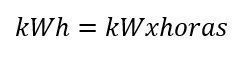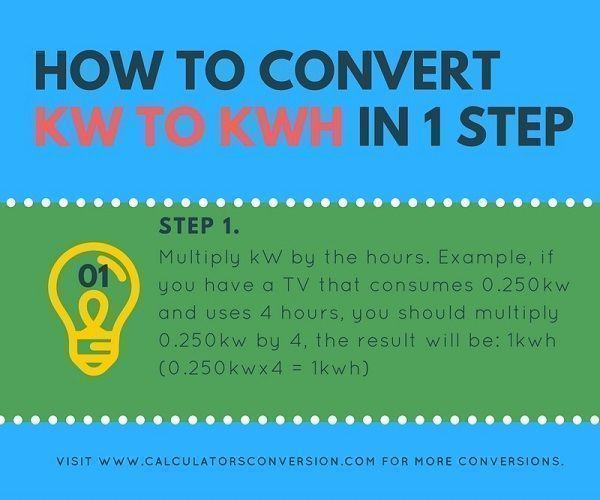# kW to kWh – Calculator, conversion, 1 phase, 2 phases, 3 phases

With this tool you can convert online from kW to kWh automatically, easy, fast and free.

For greater ease we explain that formula is used for the calculation and a table with the main conversions of kW to kWh in conversions from kW to kWh.

## kW to kWh calculation formula 3phase, 2 phase, 1 phase:• kW=kiloWatts.
• kWh=kilowatt-hours.

## How to convert kW to kWh in only 1 step.## Step 1:

Multiply kW by the hours. Example, if you have a TV that consumes 0.250kw and uses 4 hours, you should multiply 0.250kw by 4, the result will be: 1kwh (0.250kwx4 = 1kwh)

## Definition kW and kWh:

KW: is Working Power (also called Actual Power or Active Power or Real Power). It is the power that actually powers the equipment and performs useful work.

The real power in watts is the power that performs work or generates heat. Power in watts is the rate at which energy is consumed (or generated). One watt is one joule (energy) per second (1 W = 1 J/s).

Resistive devices or loads such as heaters, incandescent lamps are rated in KW.

kWh: The term kilowatt hour is a term of consumption and is defined to see the power used over a period of time, in this case one hour.

The kilowatt (kW) measures only power, kilowatt hour (kWh).

## What is the difference between a kW and a kWh?

Well, the difference is really very simple. Though it only seems simple after you understand it.

kWh is a measure of energy, whilst kW is a measure of power…

What is energy?

Electricity and other fuels supply energy in a form that we can use to run the equipment in our buildings.

The relationship between energy consumption (kWh) and time

A typical building uses more energy over long periods of time than it does over short periods of time:

• On February 16th 2010 a building might have used 95 kWh.
• Over the week starting April 12th 2010 it might have used 550 kWh.
• From January 1st 2009 to December 31st 2009 it might have used 31,250 kWh.

Given the three figures above, we can easily see that the building used more energy over the course of 2009 than it did on February 16th 2010. No surprises there.

However, we can’t immediately compare the efficiency of the building over each of those periods. If a kWh figure covers a day, we can only compare it fairly with other kWh figures that cover a day. If a kWh figure covers a week, we can only fairly compare it with other kWh figures that cover a week.

If we have the kWh from February and the kWh from March, we can’t really compare the two figures fairly, because February is typically 28 days long, whilst March is 31 days long.

Energy consumption expressed in terms of kWh doesn’t often mean much unless you also know the length of the period that the kWh were measured over. And it’s difficult to make fair comparisons between kWh figures unless they are all from periods of exactly the same length. Figures expressed in terms of power (e.g. kW) make many things more straightforward…

What is power?

Power is the rate at which energy is generated or used.

The kW is a unit of power.

(Strictly speaking energy isn’t actually generated or used, it’s converted from one form into another. Like how the energy stored in oil is converted into heat when you burn it. And like how the electricity that runs a fan is converted into the motion of the fan blade (kinetic energy). But this is a distinction that people generally don’t worry about when they’re staring at an excessive energy bill and wondering how they can “use” less energy.)

So power is a measure of how fast something is generating or using energy. The higher a building’s kW, the faster that building is using energy.

Joules per second (J/s) is a nice, clear unit of power. Joules per second makes it obvious that power is the rate at which energy is being generated or used. It’s like how miles per hour makes it obvious that speed is the rate at which distance is being travelled.

The watt (W) is another unit of power. It doesn’t make it quite so obvious what power means. But the watt is actually just another name for Joules per second. J/s and W are the same thing. Just some bright spark decided that equations and whatnot would be simpler if power had its own unit (instead of being expressed using units of energy and time together). And they named this unit after James Watt, the Scottish inventor who had an important hand in the development of the steam engine.

So, joules per second (J/s) is a measure of power… The watt (W) is a measure of power… And the kilowatt (kW) is a measure of power too (one kW being 1000 watts).

## kW to kWh table chart for conversion, equivalence, transformation:

 kW Hr kwh 1 kW 1 Hr 1 kwh 2 kW 1 Hr 2 kwh 3 kW 1 Hr 3 kwh 4 kW 1 Hr 4 kwh 5 kW 1 Hr 5 kwh 6 kW 1 Hr 6 kwh 7 kW 1 Hr 7 kwh 8 kW 1 Hr 8 kwh 9 kW 1 Hr 9 kwh 10 kW 1 Hr 10 kwh 20 kW 2 Hr 40 kwh 30 kW 2 Hr 60 kwh 40 kW 2 Hr 80 kwh 50 kW 2 Hr 100 kwh 60 kW 2 Hr 120 kwh 70 kW 2 Hr 140 kwh 80 kW 2 Hr 160 kwh 90 kW 2 Hr 180 kwh 100 kW 2 Hr 200 kwh 200 kW 3 Hr 600 kwh 300 kW 3 Hr 900 kwh 400 kW 3 Hr 1200 kwh 500 kW 3 Hr 1500 kwh 600 kW 3 Hr 1800 kwh 700 kW 3 Hr 2100 kwh 800 kW 3 Hr 2400 kwh 900 kW 3 Hr 2700 kwh 1000 kW 3 Hr 3000 kwh 2000 kW 4 Hr 8000 kwh 3000 kW 4 Hr 12000 kwh 4000 kW 4 Hr 16000 kwh 5000 kW 4 Hr 20000 kwh 6000 kW 4 Hr 24000 kwh 7000 kW 4 Hr 28000 kwh 8000 kW 4 Hr 32000 kwh 9000 kW 4 Hr 36000 kwh 10000 kW 4 Hr 40000 kwh 20000 kW 5 Hr 100000 kwh 30000 kW 5 Hr 150000 kwh 40000 kW 5 Hr 200000 kwh 50000 kW 5 Hr 250000 kwh 60000 kW 5 Hr 300000 kwh 70000 kW 5 Hr 350000 kwh 80000 kW 5 Hr 400000 kwh 90000 kW 5 Hr 450000 kwh 100000 kW 5 Hr 500000 kwh

Version en español